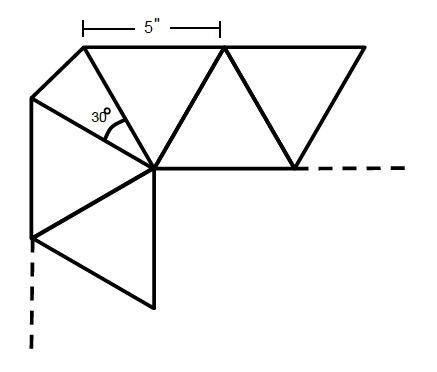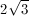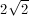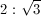## Example Questions

### Example Question #1 : How To Find The Perimeter Of An Equilateral TriangleA square rug border consists of a continuous pattern of equilateral triangles, with isosceles triangles as corners, one of which is shown above. If the length of each equilateral triangle side is 5 inches, and there are 40 triangles in total, what is the total perimeter of the rug?

The inner angles of the corner triangles is 30°.

124

180

208

200

188

188

Explanation:

There are 2 components to this problem. The first, and easier one, is recognizing how much of the perimeter the equilateral triangles take up—since there are 40 triangles in total, there must be 40 – 4 = 36 of these triangles. By observation, each contributes only 1 side to the overall perimeter, thus we can simply multiply 36(5) = 180" contribution.

The second component is the corner triangles—recognizing that the congruent sides are adjacent to the 5-inch equilateral triangles, and the congruent angles can be found by

180 = 30+2x → x = 75°

We can use ratios to find the unknown side:

75/5 = 30/y → 75y = 150 → y = 2''.

Since there are 4 corners to the square rug, 2(4) = 8'' contribution to the total perimeter. Adding the 2 components, we get 180+8 = 188 inch perimeter.

### Example Question #2 : How To Find The Perimeter Of An Equilateral Triangle

The height of an equilateral triangle isWhat is the triangle's perimeter?

86

24

12

12

Explanation:

An altitude drawn in an equilateral triangle will form two 30-60-90 triangles. The height of equilateral triangle is the length of the longer leg of the 30-60-90 triangle. The length of the equilateral triangle's side is the length of the hypotenuse of the 30-60-90.

The ratio of the length of the hypotenuse to the length of the longer leg of a 30-60-90 triangle isThe length of the longer leg of the 30-60-90 triangle in this problem isUsing this ratio, we find that the length of this triangle's hypotenuse is 4. Thus the perimeter of the equilateral triangle will be 4 multiplied by 3, which is 12.